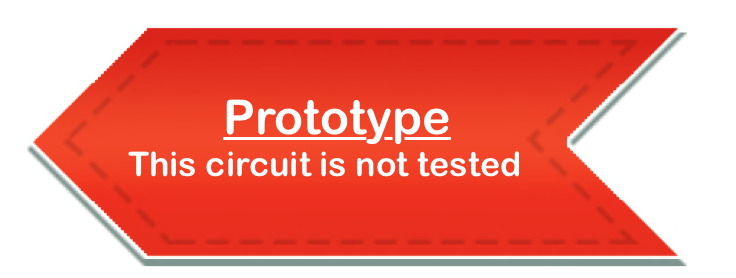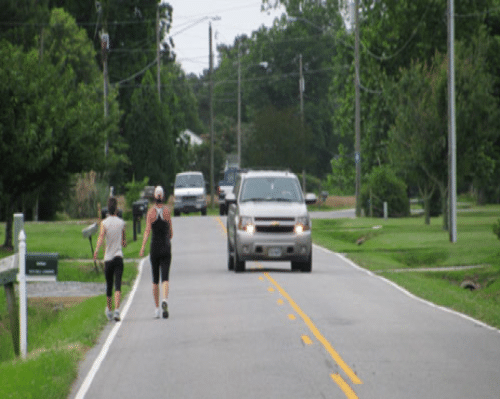Pedestrian Detection Using MATLAB

Sivakumaran is a MATLAB Programmer in Photon Technologies, Chennai. He has completed his masters in VLSI Design.

17716MATLAB (matrix laboratory)is a multi-paradigm numerical computing language. A proprietary programming language designed and developed by Math Works, MATLAB permits matrix utilization, plotting of tasks and information, implementation of algorithms, the formation of user boundaries, and interfacing with programs written in further languages, including C, C++, C#, Java, Fortran and Python.

Although MATLAB is intended chiefly for arithmetical computing, an elective toolbox uses the MuPAD symbolic engine, allowing contact with symbolic computing capabilities. An additional pack up, Simulink, adds graphical multi-field simulation and prototype-based design for vibrant and embedded systems. MATLAB is a high- accomplishment language for technological computing.

It contains facilities for managing the changeable in your workspace and introducing and exporting data. It also contains tools for growing, managing, debugging, and profiling M-files, MATLAB’s functions.

Image processing

Image processing is a technique to execute some functions on an image, in order to get an improved image or to extract some helpful information from it. Here are two kinds of methods used for image processing that is, analogue and digital image processing. Analogue image processing can be employed for the hard prints like printouts and photographs. Image psychoanalysts use various essentials of interpretation while using these image techniques. Digital image processing methods help in the operation of the digital images by using computers. The three common phases that all kinds of data have to undergo while using digital method are pre-processing, improvement, and display, Usually Image Processing system contains treating images as two-dimensional gestures. the input is an image and the output is also an image is complete in image processing. As it name proposes, it deals with the dispensation on images.What is an Image?

An image is nothing more than a two-dimensional gesture. It is defined by the arithmetical function f(x,y) where x and y are the two co-ordinates parallel and vertically.
The value of f(x,y) at any point provides the pixel value at that point of a picture.

Pedestrian detection

Project for driverless car project:

Pedestrians are the most vulnerable road users, whilst also being the most tricky to observe both in a day and in night situations. Pedestrians in the vehicle trail or walking into the vehicle trail are at risk of being hit causing severe injury both to the pedestrian and potentially also to the vehicle inhabitants. Pedestrian recognition is an essential and important task in any intelligent video observation system, as it gives the essential information for the semantic understanding of the video copies. It has an obvious extension to automotive appliances due to the potential for enhancing security systems.

%% Compute Distance among camera and Pedestrian

num_people=length(scores);
mx_distance=max(scores);
meter=30*3;
distanc_frm_vechl=meter*mx_distance;
bboxA = [150,80,100,100];
bboxB = bboxA + 50;
overlapRatio = OverlapRatio(bboxA,bboxB)
bboxA = 10*rand(5,4);
bboxB = 10*rand(10,4);
bboxA(:,3:4) = bboxA(:,3:4) + 10;
bboxB(:,3:4) = bboxB(:,3:4) + 10;
overlapRatio = OverlapRatio(bboxA,bboxB)

if is empty(distanc_frm_vechl);
h=msgbox(‘Error Data base’);
else
bbxcount=size(bboxes)
bbx=bbxcount(1)
axes(handles.axes3)
imshow(I)
hold on
j=1;
for i=1:bbx

rectangle(‘Position’,bboxes(j,:),’EdgeColor’,’r’,’LineWidth’,2)
j=j+1
title(‘Pedestrian Detected’)%% Pedestrian detection scheme model using Machine Learning Algorithm
% with SVM Proposed Model
% extract HOG features
[a, b]=uigetfile(‘*.jpg’);
% figure,imshow(a)
pedstrain_tracking = vision.PeopleDetector;
% I = imread(‘visionteam1.jpg’);
I=a;
imgdims=ndims(a);
[m n c]=size(a);
datah=582;
dataw=800;
h=isequal(datah,m)
w=isequal(dataw,n)
I=imresize(I,[datah dataw]);
axes(handles.axes1)
imshow(I)
title(‘Input Image’)
grayimg=rgb2gray(I);

People Detector describes and set up your people detector article using the constructor.
Call the step process with the input image, I, and the persons detector object, persons Detector. See the syntax below for using the step technique.

BBOXES = step(persons Detector,I) performs multi scale object detection on the input image, I. The technique returns an M-by-4 matrix defining M bounding boxes, where M symbolizes the number of detected people. Every row of the output matrix, BBOXES, includes a four-element vector, [x y width height]. This vector specifies, in pixels, the upper-left corner and size, of a bouncing box. When no persons are detected, the step process returns a blank vector. The input image, I, should be a grayscale or true color (RGB) image.

[BBOXES,SCORES]= step(people Detector, I) returns a trust value for the detections. The M-by-1 vector, SCORES, enclose positive values for every bouncing box in BBOXES. Larger score values point out a higher trust in the detection. The SCORES value depends on how you set the Merge Detections property. When you set the property to accurate, the people detector algorithm estimates categorization results to produce the SCORES value. When you set the property to fake, the detector returns the unchanged categorization SCORES.
[___] = step(people Detector ,I, roi) notices people within the rectangular search section specified by roi. You must identify roi as a 4-element vector, [x y width height], that describes a rectangular section of interest within image I. Set the ‘Use ROI’ property to real to use this syntax.

rectangle(‘Position’,pos)
I=insertObjectAnnotation(I,’rectangle’,bboxes,scores);
figure, imshow(I)
title(‘Detected people and detection scores’);

%Detect objects using Viola-Jones Algorithm
global I
I=imresize(I,[256 256]);
%To detect Face

%Returns Bounding Box values based on number of objects
BB = step(FDetect,I);
figure,
imshow(I); hold on
for i = 1:size(BB,1)
rectangle(‘Position’,BB(i,:),’LineWidth’,5,’LineStyle’,’-‘,’EdgeColor’,’r’);
end